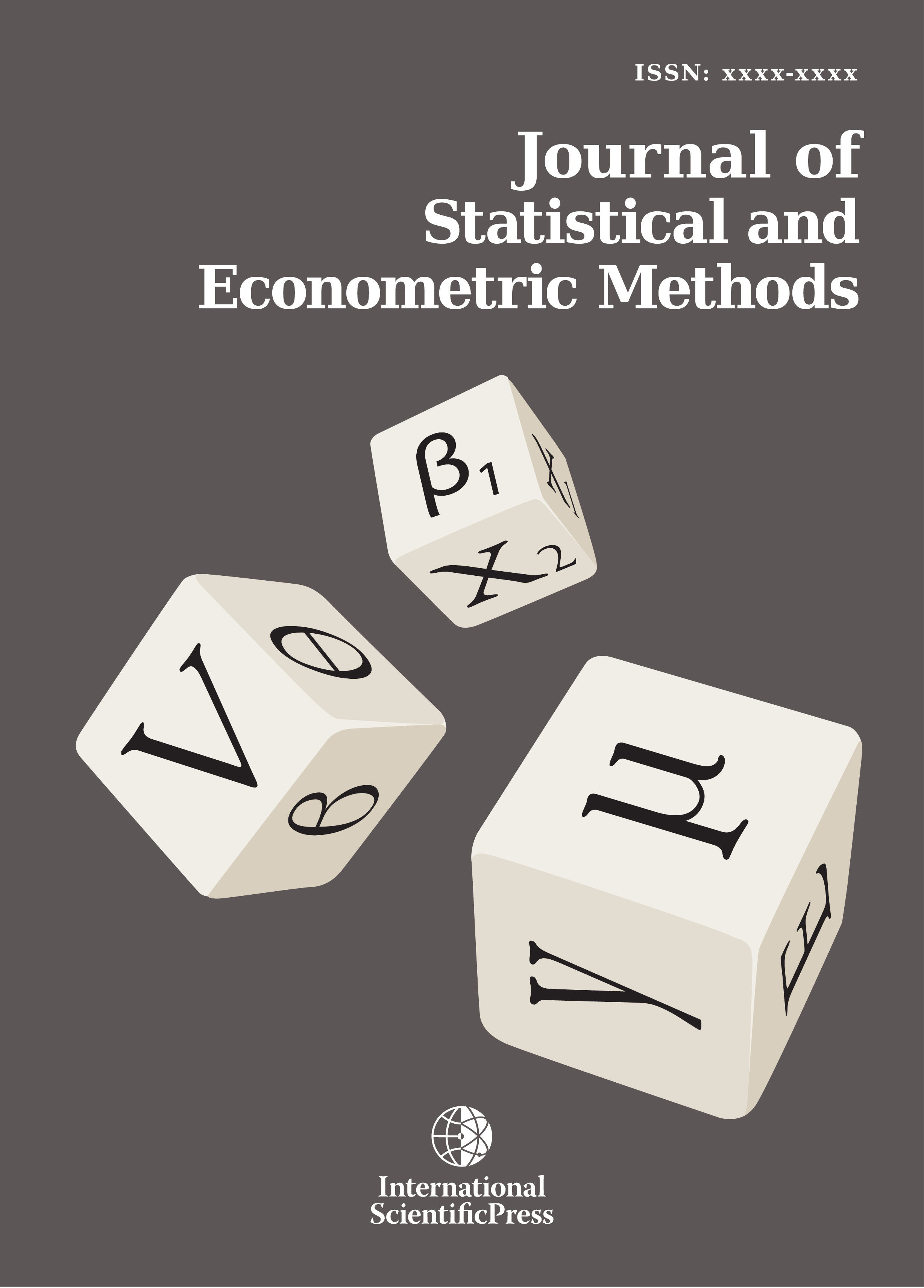# Journal of Statistical and Econometric Methods

#### Two and Three Stage Least Squares as Aitken estimators

•[ Download ]
• Abstract

This note generalizes the existing derivation of Two and Three Stage Least Squares estimators as Aitken Generalised Least Squares estimators.

JEL classification numbers: C01, C13, C30.

Keywords: Two Stage Least Squares estimators, Three Stage Least Squares estimators, Generalized Least Squares estimators, Aitken estimators.ISSN: 2241-0376 (Online)
2241-0384 (Print)